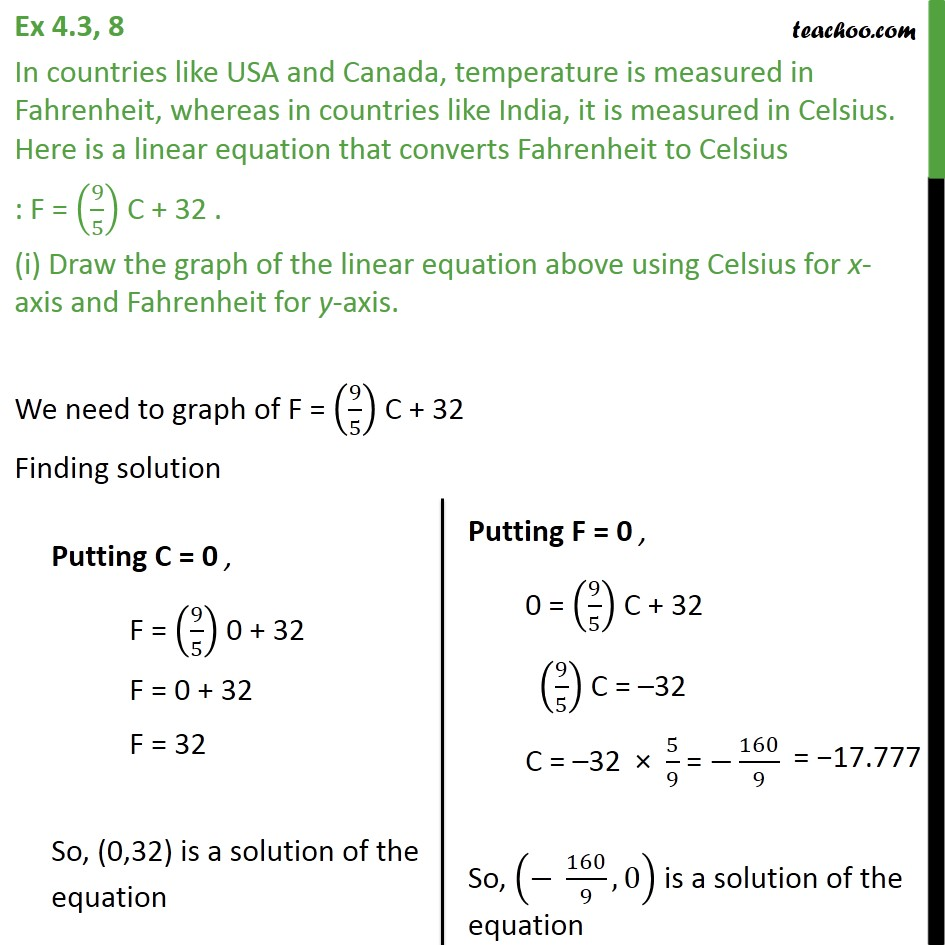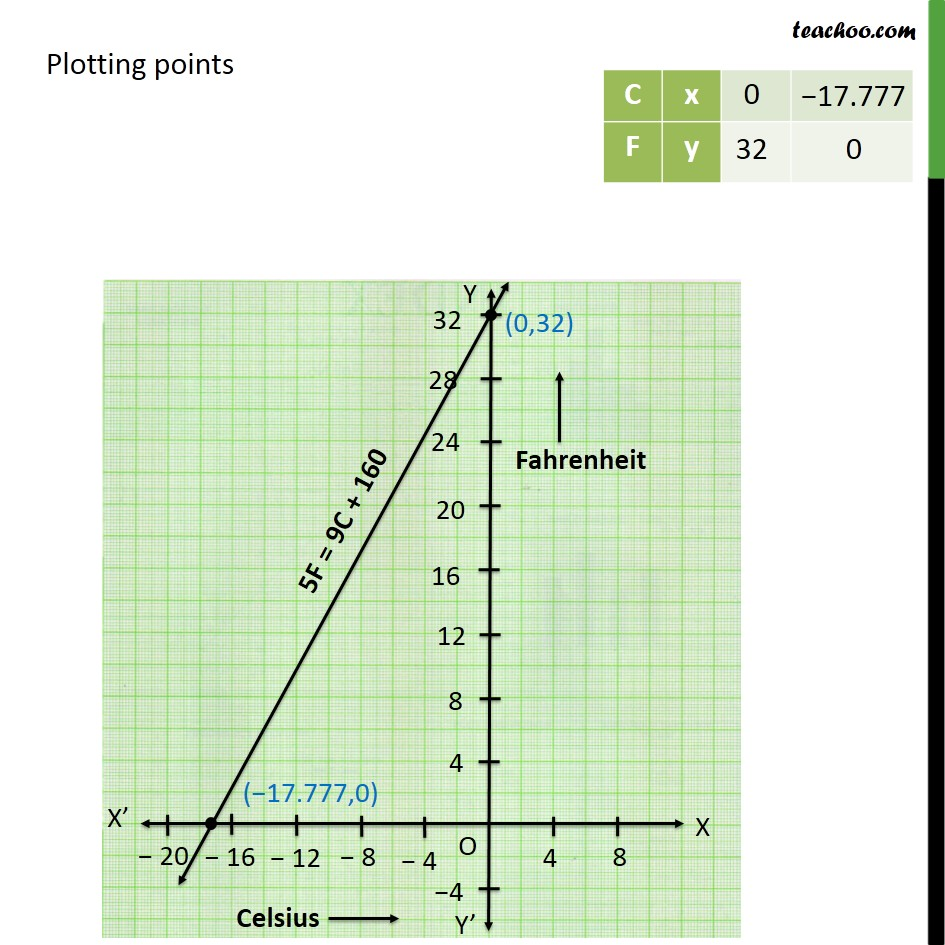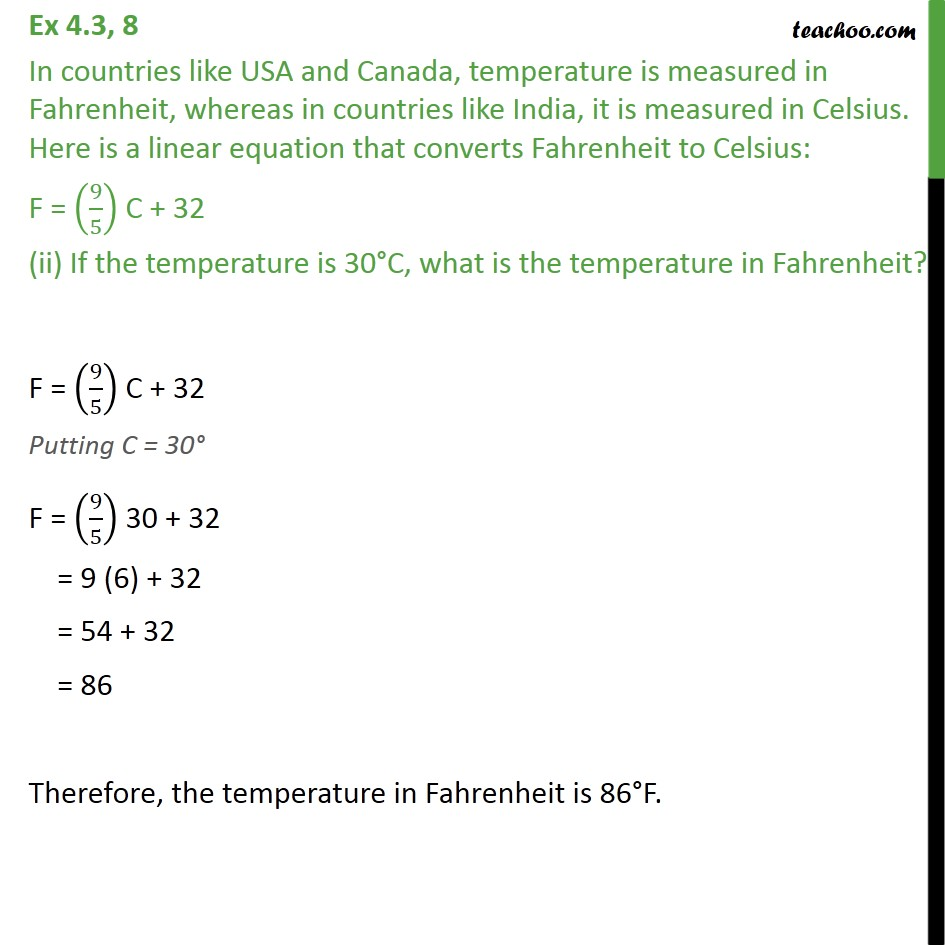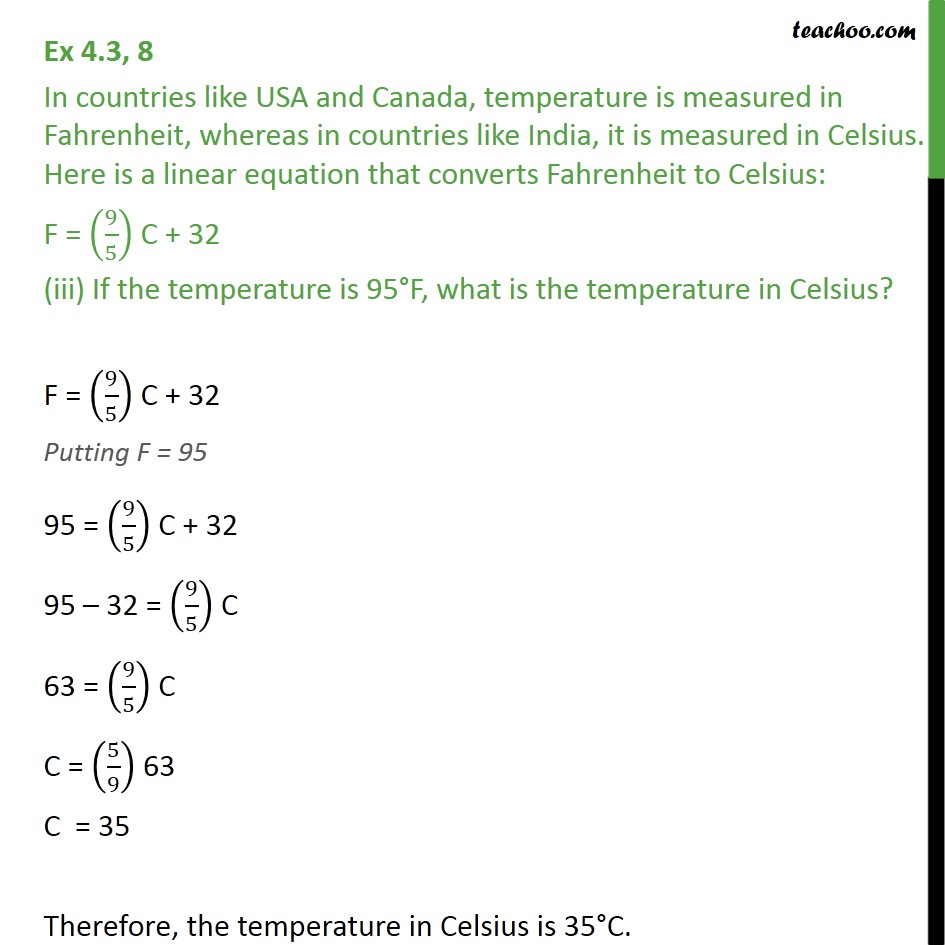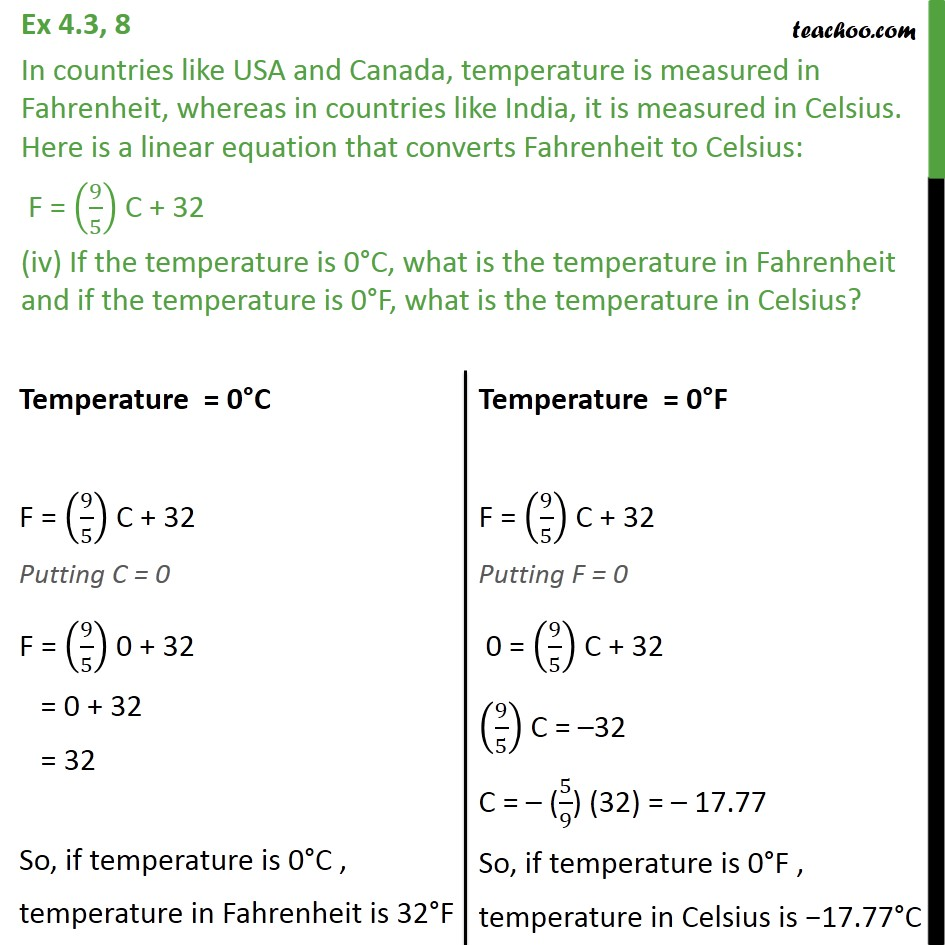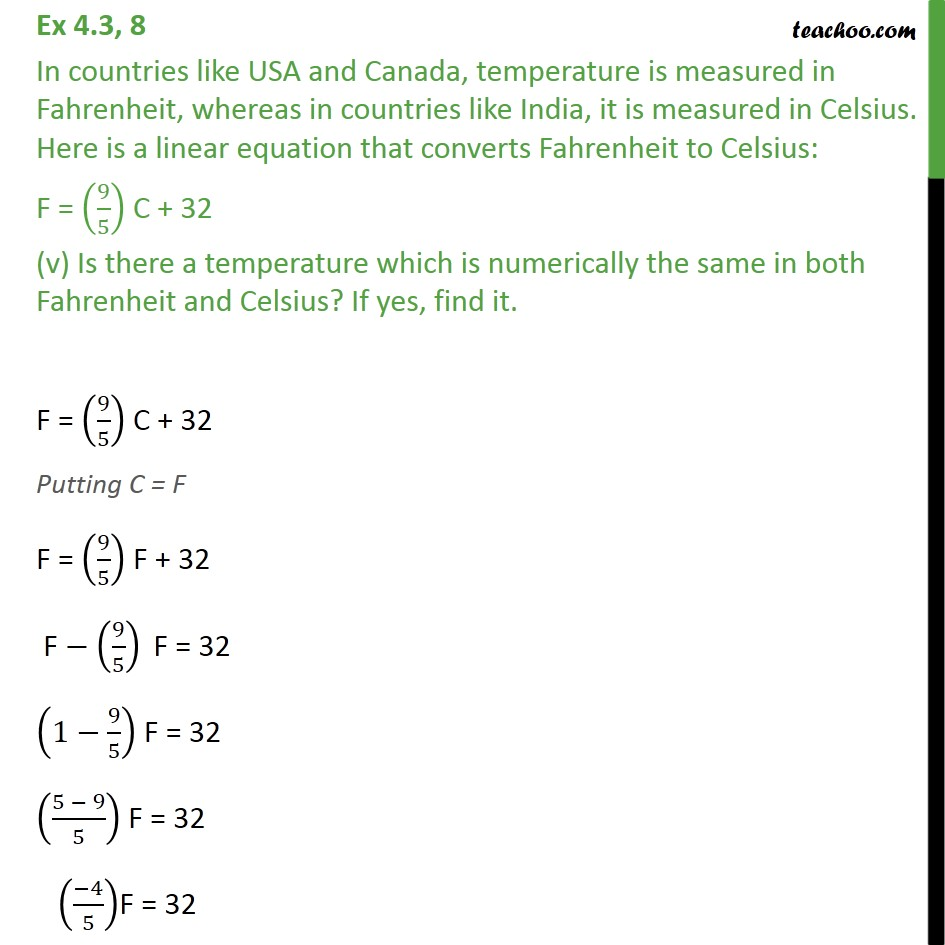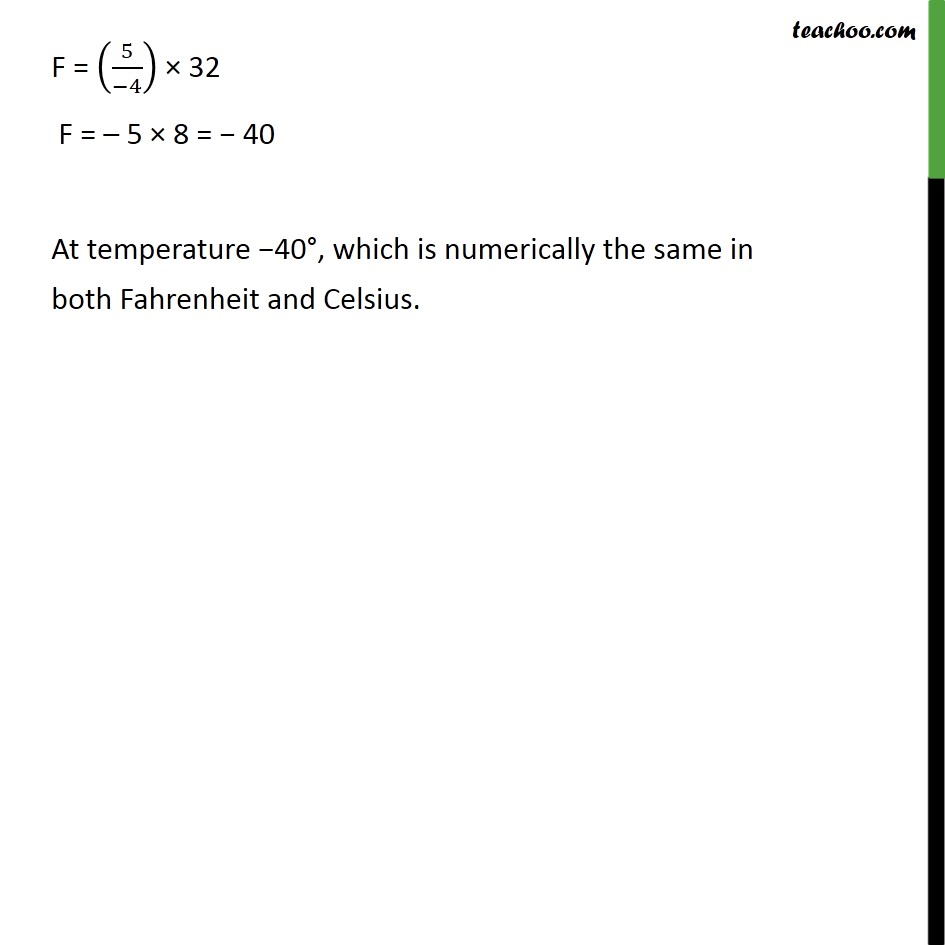1. Chapter 4 Class 9 Linear Equations in Two Variables
2. Concept wise
3. Graph of linear equations

Transcript

Ex 4.3, 8 In countries like USA and Canada, temperature is measured in Fahrenheit, whereas in countries like India, it is measured in Celsius. Here is a linear equation that converts Fahrenheit to Celsius : F = (9/5) C + 32 . (i) Draw the graph of the linear equation above using Celsius for x-axis and Fahrenheit for y-axis. We need to graph of F = (9/5) C + 32 Finding solution Plotting points Ex 4.3, 8 In countries like USA and Canada, temperature is measured in Fahrenheit, whereas in countries like India, it is measured in Celsius. Here is a linear equation that converts Fahrenheit to Celsius: F = (9/5) C + 32 (ii) If the temperature is 30 C, what is the temperature in Fahrenheit? F = (9/5) C + 32 Putting C = 30 F = (9/5) 30 + 32 = 9 (6) + 32 = 54 + 32 = 86 Therefore, the temperature in Fahrenheit is 86 F. Ex 4.3, 8 In countries like USA and Canada, temperature is measured in Fahrenheit, whereas in countries like India, it is measured in Celsius. Here is a linear equation that converts Fahrenheit to Celsius: F = (9/5) C + 32 (iii) If the temperature is 95 F, what is the temperature in Celsius? F = (9/5) C + 32 Putting F = 95 95 = (9/5) C + 32 95 32 = (9/5) C 63 = (9/5) C C = (5/9) 63 C = 35 Therefore, the temperature in Celsius is 35 C. Ex 4.3, 8 In countries like USA and Canada, temperature is measured in Fahrenheit, whereas in countries like India, it is measured in Celsius. Here is a linear equation that converts Fahrenheit to Celsius: F = (9/5) C + 32 (iv) If the temperature is 0 C, what is the temperature in Fahrenheit and if the temperature is 0 F, what is the temperature in Celsius? Ex 4.3, 8 In countries like USA and Canada, temperature is measured in Fahrenheit, whereas in countries like India, it is measured in Celsius. Here is a linear equation that converts Fahrenheit to Celsius: F = (9/5) C + 32 (v) Is there a temperature which is numerically the same in both Fahrenheit and Celsius? If yes, find it. F = (9/5) C + 32 Putting C = F F = (9/5) F + 32 "F" (9/5)" F "= 32 (1 9/5) F = 32 ((5 9)/5) F = 32 (( 4)/5)F = 32 F = (5/( 4)) 32 F = 5 8 = 40 At temperature 40 , which is numerically the same in both Fahrenheit and Celsius.

Graph of linear equations